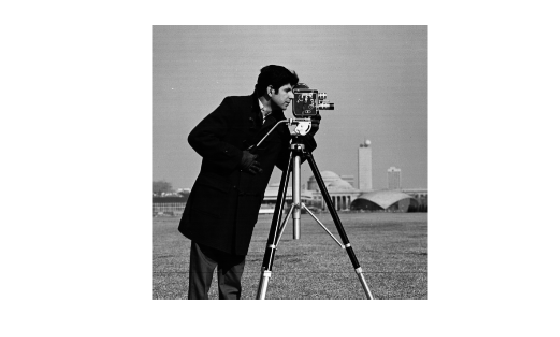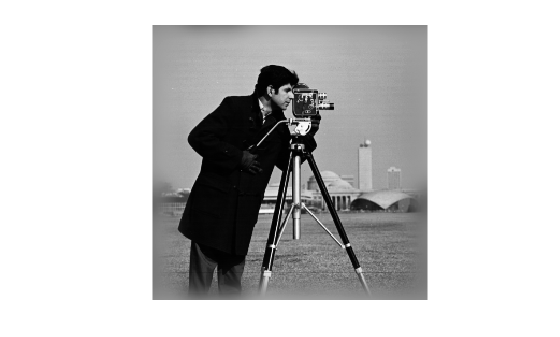# edgetaper

Taper discontinuities along image edges

## Syntax

``J = edgetaper(I,PSF)``

## Description

example

````J = edgetaper(I,PSF)` blurs the edges of the input image `I` using the point spread function `PSF`. The output image `J` is the weighted sum of the original image `I` and its blurred version. The weighting array, determined by the autocorrelation function of `PSF`, makes `J` equal to `I` in its central region, and equal to the blurred version of `I` near the edges.The `edgetaper` function reduces the ringing effect in image deblurring methods that use the discrete Fourier transform, such as `deconvwnr`, `deconvreg`, and `deconvlucy`.```

## Examples

collapse all

```original = imread('cameraman.tif'); PSF = fspecial('gaussian',60,10); edgesTapered = edgetaper(original,PSF); figure, imshow(original,[]);````figure, imshow(edgesTapered,[]);`## Input Arguments

collapse all

Input image, specified as a numeric array.

Data Types: `single` | `double` | `int16` | `uint8` | `uint16`

Point spread function, specified as a numeric array. The size of the `PSF` cannot exceed half of the image size in any dimension.

Data Types: `single` | `double` | `int16` | `uint8` | `uint16`

## Output Arguments

collapse all

Weighted sum of original image and its blurred version, returned as a numeric array the same size and class as `I`. The weighting array, determined by the autocorrelation function of `PSF`, makes `J` equal to `I` in its central region, and equal to the blurred version of `I` near the edges.